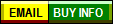CLICK ABOVE to see the
power to weight ration
acceleration comparisonsSo, how cost effective is the Performance of a Superformance? Take some of the greatest road cars ever built, put them all together side by side and compare which gets the most "bang for the buck!" The ability to accelerate a given mass, a given distance in the shortest period of time is a simple mathematical calculation expressed in Pounds per Horsepower, more commonly called the "Power to Weight Ratio." Now take that number and consider in the cost of the automobile, and you have a general comparison of how much performance your getting for your dollar. Keep in mind this comparison does not reflect the value, styling or accessories of a car's worth, just an entertaining look at what  all could be comparably as much fun for the same dollar. The chart below graphically shows how the Mark-III stands up to some of the greatest cars ever built…formula used =   10 / (weight ratio x 2) x (cost / 20000)
...this formula places an increased emphasis on the power and acceleration, and a secondary of the cost of the package